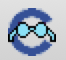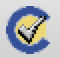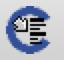# CiaoPP at a glance

Author(s): Isabel Garcia-Contreras, Daniela Ferreiro.

The purpose of this document is to quickly illustrate some of the kinds of static processing that CiaoPP can perform. Note that this is not intended to be a tutorial, for that please follow A Gentle Introduction to Static Verification and Bug Finding with CiaoPP or Program Development using the CiaoPP Program Processor.

## Setup

In order to follow these examples you need to either:

• Install Ciao on your computer, including the development environment (see Installation for more information). This includes CiaoPP (and LPdoc). You can then access CiaoPP from the Emacs interface or the command line.

• Run CiaoPP directly on your browser through the Ciao playground. To this end, load the examples into the playground by pressing the button (``Load in playground''), and then CiaoPP can be run clicking the More... button and selecting Analyze and check assertions.

The instructions below use the Emacs interface but the steps can also be performed in the playground version.

## Analyzing

Let us analyze this implementation of the append predicate:

```:- module(_, [app/3], [assertions]).

:- pred app(A,B,C) : (list(A), list(B)) => var(C).

app([],Y,Y).
app([X|Xs], Ys, [X|Zs]) :-
app(Xs,Ys,Zs).```
Automatic analysis can be performed by using the direct access button in the Emacs interface.

By default, CiaoPP analyzes programs with a type domain (eterms) and a sharing/freeness (modes) domain (shfr). We can open the file with the inferred information by typing C-c C-v.

The inferred information is expressed with (true) assertions (for a more extensive tutorial of the assertion language see Using assertions for preprocessing programs). Assertion marked as true contain safe approximations (safe abstractions) of the behavior of the program that were inferred by the analyzer. In this case CiaoPP inferred:

```:- true pred app(A,B,C)
: mshare([[A],[A,B],[A,B,C],[A,C],[B],[B,C],[C]])
=> mshare([[A,B,C],[A,C],[B,C]]).

:- true pred app(A,B,C)
: ( list(A), list(B), term(C) )
=> ( list(A), list(B), list(C) ).```

The first assertion contains type information and expresses the fact that predicate app/3 is called (: field) with A and B bound to lists and, if it succeeds (=> field), then C will be a list.

The second assertion contains information about how variables are shared. It was inferred that when app(A,B,C) is called arguments A, B, and C may be shared and if it succeeds they also will be shared, sice C is the concatenation of A and B.

## Assertion Checking

In the example above, we have also added an assertion with properties that we want to prove about the execution of the program.

`:- pred app(A,B,C) : (list(A), list(B)) => var(C).`
This assertion is stating that if predicate append/3 is called with A and B bound to lists, then, if the call succeeds, C will be a free variable. This is not true in general of course. We can check this assertion by clicking theicon in the Emacs interface and we obtain:

```ERROR (ctchecks_pred_messages): (lns 3-3) False assertion:
:- check success app(A,B,C)
: ( list(A), list(B) )
=> var(C).
because the success field is incompatible with inferred success:
[eterms] basic_props:list(A),basic_props:list(B),basic_props:list(C)

ERROR (auto_interface): Errors detected. Further preprocessing aborted.```

Since this assertion does not hold we get a message saying so.

Assertion checking can also be reported within the source code. If we open the output file the CiaoPP produces (C-c C-v), we see that the analyzer proves the assertion that we had included false:

```:- checked calls app(A,B,C)
: ( list(A), list(B) ).

:- false success app(A,B,C)
: ( list(A), list(B) )
=> var(C).```

So we correct it with:

`:- pred app(A,B,C) : (list(A), list(B)) => list(C).`
and run the analysis again. In this case we get no error messages and, as before, results can also be reported in the source file:

```:- checked calls app(A,B,C)
: ( list(A), list(B) ).

:- checked success app(A,B,C)
: ( list(A), list(B) )
=> list(C).```

## Program Optimization

The following program naively implements a predicate that duplicates the first element of a list.

```:- module(_, [dup_first/2], []).

dup_first([X|Xs], Zs) :-
app([X], [X|Xs], Zs).

app([],Y,Y).
app([X|Xs], Ys, [X|Zs]) :-
app(Xs,Ys,Zs). ```
CiaoPP can automatically optimize sources by using thebutton:

```:- module(_1,[dup_first/2],[assertions]).

dup_first([A|B],[A,A|B]).```